# cranioid

## trigonometric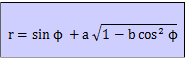The cranioid resembles a skull 1). An alternative name is: eyeball curve. The curve is a quartic 2) curve. Some examples of the curve: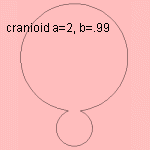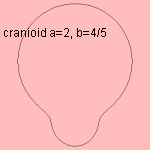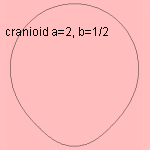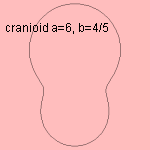It occurs that authors add a term c √ (1 - d cos2 φ) to the polar formula of the curve. In my opinion this adds obsolete complexity and parameters to the formula. notes 1) From the Greek word κρανιο that means skull. 2) With equation: (x2 + y2)2 - 2 y2 (y + x) + a2 (b - 1) x2 + (1 - a2) x2 = 0.